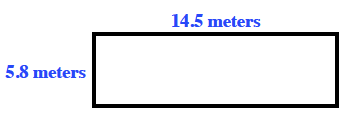### Home > CC1MN > Chapter 8 > Lesson 8.1.4 > Problem8-53

8-53.

Find the area and perimeter of a rectangle that is $14.5$ meters by $5.8$ meters.

Below is a sketch of the rectangle described in this problem.
Use this diagram if it helps you with finding the area and the perimeter.Remember that area is the space within a shape and perimeter is the distance around a shape.